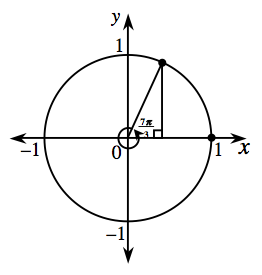### Home > INT3 > Chapter 9 > Lesson 9.1.7 > Problem9-88

9-88.

What is the measure in degrees of a central angle measuring $\frac { 7 \pi } { 3 }$ radians?

$\text{What number of degrees is equivalent to }\frac{\pi}{3}\text{ radians?}$

If you can't remember, calculate.

$\pi=180^\circ \ \ \ \ \ \ \ \ \frac{\pi}{3} = 60^\circ$

Now multiply the angle by $7$.

$420^\circ$

1. What other angles correspond to the same point on the circle?

The distance around the unit circle is $2π$, no matter what point you start from.

$\frac{\pi}{3} \:\pm\:2\pi n$

2. Make a sketch of the unit circle showing the resulting right triangle.

The angle you are working with, $420^\circ$, is more than $360^\circ$. How much more?3. What are sin$( \frac { 7 \pi } { 3 } )$, cos$( \frac { 7 \pi } { 3 } )$ and tan$( \frac { 7 \pi } { 3 } )$ exactly?

Notice that the triangle formed is a $30^\circ-60^\circ-90^\circ$ triangle.

$\text{sin}(\frac{7\pi}{3})=\frac{\sqrt{3}}{2}$                       $\text{cos}(\frac{7\pi}{3})=\frac{1}{2}$                      $\text{tan}(\frac{7\pi}{3})={\sqrt{3}}$